Publication date: 08/13/2020

## Stepwise Regression Control Panel

Use the Stepwise Regression Control panel to limit regressor effect probabilities, determine the method of selecting effects, begin or stop the selection process, and run a model. A note appears beneath the Go button to indicate whether you have excluded or missing rows.

Figure 5.3 Stepwise Regression Control Panel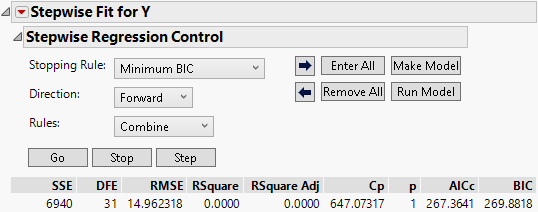### Stopping Rule

The Stopping Rule determines which model is selected. For all stopping rules other than P-value Threshold, only the Forward and Backward directions are allowed. The only stopping rules that use validation are Max Validation RSquare and Max K-Fold RSquare. See Using Validation.

P-value Threshold

Uses p-values (significance levels) to enter and remove effects from the model. Two other options appear when you choose P-value Threshold:

Prob to Enter

Specifies the maximum p-value that an effect must have to be entered into the model during a forward step.

Prob to Leave

Specifies the minimum p-value that an effect must have to be removed from the model during a backward step.

Minimum AICc

Uses the minimum corrected Akaike Information Criterion to choose the best model. For more details, see Likelihood, AICc, and BIC in the Statistical Details section.

Minimum BIC

Uses the minimum Bayesian Information Criterion to choose the best model. For more details, see Likelihood, AICc, and BIC in the Statistical Details section.Max Validation RSquare

Uses the maximum R-square from the validation set to choose the best model. This is available only when you use a validation column with two or three distinct values. For more information about validation, see Validation Set with Two or Three Values.

Max K-Fold RSquare

Uses the maximum RSquare from K-fold cross validation to choose the best model. You can access the Max K-Fold RSquare stopping rule by selecting this option from the Stepwise red triangle menu. JMP Pro users can access the option by using a validation set with four or more values. When you select this option, you are asked to specify the number of folds. For more information about validation, see K-Fold Cross Validation.

### Direction

The Direction you choose controls how effects enter and leave the model. Select one of the following options:

Forward

Enters the term with the smallest p-value. If the P-value Threshold stopping rule is selected, that term must be significant at the level specified by Prob to Enter. See Forward Selection Example.

Backward

Removes the term with the largest p-value. If the P-value Threshold stopping rule is selected, that term must not be significant at the level specified in Prob to Leave. See Backward Selection Example.

Note: When Backward is selected as the Direction, you must click Enter All before clicking Go or Step.

Mixed

Available only when the P-value Stopping Rule is selected. It alternates the forward and backward steps. It includes the most significant term that satisfies Prob to Enter and removes the least significant term satisfying Prob to Leave. It continues removing terms until the remaining terms are significant and then it changes to the forward direction.

### Go, Stop, Step Buttons

The Go, Stop, and Step buttons enable you to control how terms are entered or removed from the model.

Note: All Stopping Rules consider only models defined by p-value entry (Forward direction) or removal (Backward direction). Stopping rules do not consider all possible models.

Go

Automates the process of entering (Forward direction) or removing (Backward direction) terms. Among the fitted models, the model that is considered best based on the selected Stopping Rule is listed last. Except for the P-value Threshold stopping rule, the model selected as Best is one that overlooks local dips in the behavior of the stopping rule statistic. The button to the right the Best model selects it for the Make Model and Run Model options, but you are free to change this selection.

For P-value Threshold, the best model is based on the Prob to Enter and Prob to Leave criteria. See P-value Threshold.

For Min AICc and Min BIC, the automatic fits continue until a Best model is found. The Best model is one with a minimum AICc or BIC that can be followed by as many as ten models with larger values of AICc or BIC, respectively. This model is designated by the terms Best in the Parameter column and Specific in the Action column.

For Max Validation RSquare (JMP Pro only) and Max K-Fold RSquare, the automatic fits continue until a Best model is found. The Best model is one with an RSquare Validation or RSquare K-Fold value that can be followed by as many as ten models with smaller values of RSquare Validation or RSquare K-Fold, respectively. This model is designated by the terms Best in the Parameter column and Specific in the Action column.

Stop

Stops the automatic selection process started by the Go button.

Step

Enters terms one-by-one in the Forward direction or removes them one-by one in the Backward direction. At any point, you can select a model by clicking its button on the right in the Step History report. The selection of model terms is updated in the Current Estimates report. This is the model that is used once you click Make Model or Run Model.

### Rules

Note: Appears only if your model contains related terms. When you have a nominal or ordinal variable, related terms are constructed and appear in the Current Estimates table.

Use Rules to change the rules that are applied when there is a hierarchy of terms in the model. A hierarchy can occur in the following ways:

A hierarchy results when a variable is a component of another variable. For example, if your model contains variables A, B, and A*B, then A and B are precedent terms to A*B in the hierarchy.

A hierarchy also results when you include nominal or ordinal variables. A term that is above another term in the tree structure is a precedent term. See Construction of Hierarchical Terms.

Select one of the following options:

Combine

Calculates p-values for two separate tests when considering entry for a term that has precedents. The first p-value, p1, is calculated by grouping the term with its precedent terms and testing the group’s significance probability for entry as a joint F test. The second p-value, p2, is the result of testing the term’s significance probability for entry after the precedent terms have already entered into the model. The final significance probability for entry for the term that has precedents is max(p1, p2).

Tip: The Combine rule avoids including non-significant interaction terms, whose precedent terms can have particularly strong effects. In this scenario, the strong main effects might make the group’s significance probability for entry, p1, very small. However, the second test finds that the interaction by itself is not significant. As a result, p2 is large and is used as the final significance probability for entry.

Caution: The degrees of freedom value for a term that has precedents depends on which of the two significance probabilities for entry is larger. The test used for the final significance probability for entry determines the degrees of freedom, nDF, in the Current Estimates table. Therefore, if p1 is used, nDF equals the number of terms in the group for the joint test, and if p2 is used, nDF equals 1.

The Combine option is the default rule. See Models with Crossed, Interaction, or Polynomial Terms.

Restrict

Restricts the terms that have precedents so that they cannot be entered until their precedents are entered. See Models with Nominal and Ordinal Effects and Example of the Restrict Rule for Hierarchical Terms.

No Rules

Gives the selection routine complete freedom to choose terms, regardless of whether the routine breaks a hierarchy or not.

Whole Effects

Enters only whole effects, when terms involving that effect are significant. This rule applies only when categorical variables with more than two levels are entered as possible model effects. See Rules.

### Buttons

The Stepwise Control Panel contains the following buttons:

Go

Automates the selection process to completion.

Stop

Stops the selection process.

Step

Increments the selection process one step at a time.

Arrow buttons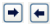Step forward and backward one step in the selection process.

Enter All

Enters all unlocked terms into the model.

Remove All

Removes all unlocked terms from the model.

Make Model

Creates a model for the Fit Model window from the model currently showing in the Current Estimates table. In cases where there are nominal or ordinal terms, Make Model creates temporary transform columns that contain terms that are needed for the model.

Run Model

Runs the model currently showing in the Current Estimates table. In cases where there are nominal or ordinal terms, Run Model creates temporary transform columns that contain terms that are needed for the model.

### Statistics

The following statistics appear below the Stepwise Regression Control panel.

SSE

Sum of squared errors for the current model.

DFE

Error degrees of freedom for the current model.

RMSE

Root mean square error (residual) for the current model.

RSquare

Proportion of the variation in the response that can be attributed to terms in the model rather than to random error.

Adjusts R2 to make it more comparable over models with different numbers of parameters by using the degrees of freedom in its computation. The adjusted R2 is useful in stepwise procedure because you are looking at many different models and want to adjust for the number of terms in the model.

Cp

Mallow’s Cp criterion for selecting a model. It is an alternative measure of total squared error and can be defined as follows: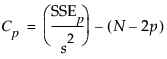where s2 is the MSE for the full model and SSEp is the sum-of-squares error for a model with p variables, including the intercept. Note that p is the number of x-variables+1. If Cp is graphed with p, Mallows (1973) recommends choosing the model where Cp first approaches p.

p

Number of parameters in the model, including the intercept.

AICc

Corrected Akaike’s Information Criterion. For more details, see Likelihood, AICc, and BIC in the Statistical Details section.

BIC

Bayesian Information Criterion. For more details, see Likelihood, AICc, and BIC in the Statistical Details section.

### Forward Selection Example

In forward selection, terms are entered into the model and most significant terms are added until all of the terms are significant.

1. Complete the steps in Example Using Stepwise Regression.

Notice that the default selection for Direction is Forward.

2. Click Step.

In Figure 5.4, you can see that after one step, the most significant term, Runtime, is entered into the model.

3. Click Go.

In Figure 5.5 you can see that all of the terms have been added, except RstPulse and Weight.

Figure 5.4 Current Estimates Table for Forward Selection after One Step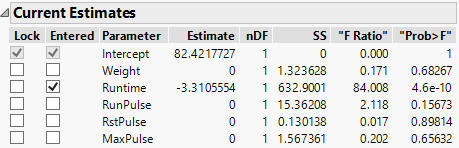Figure 5.5 Current Estimates Table for Forward Selection after Three Steps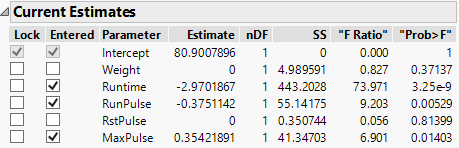### Backward Selection Example

In backward selection, all terms are entered into the model and then the least significant terms are removed until all of the remaining terms are significant.

1. Complete the steps in Example Using Stepwise Regression.

2. Click Enter All.

Figure 5.6 All Effects Entered into the Model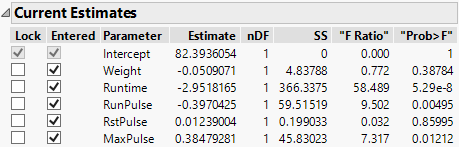3. For Direction, select Backward.

4. Click Step two times.

The first backward step removes RstPulse and the second backward step removes Weight.

Figure 5.7 Current Estimates with Terms Removed and Step History Table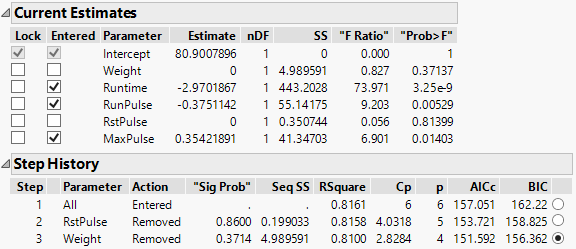The Current Estimates and Step History tables shown in Figure 5.7 summarize the backward stepwise selection process. Note the BIC value of 156.362 for the third step in the Step History table. If you click Step again to remove another parameter from the model, the BIC value increases to 159.984. For this reason, you choose the step 3 model. This is also the model that the Go button produces.